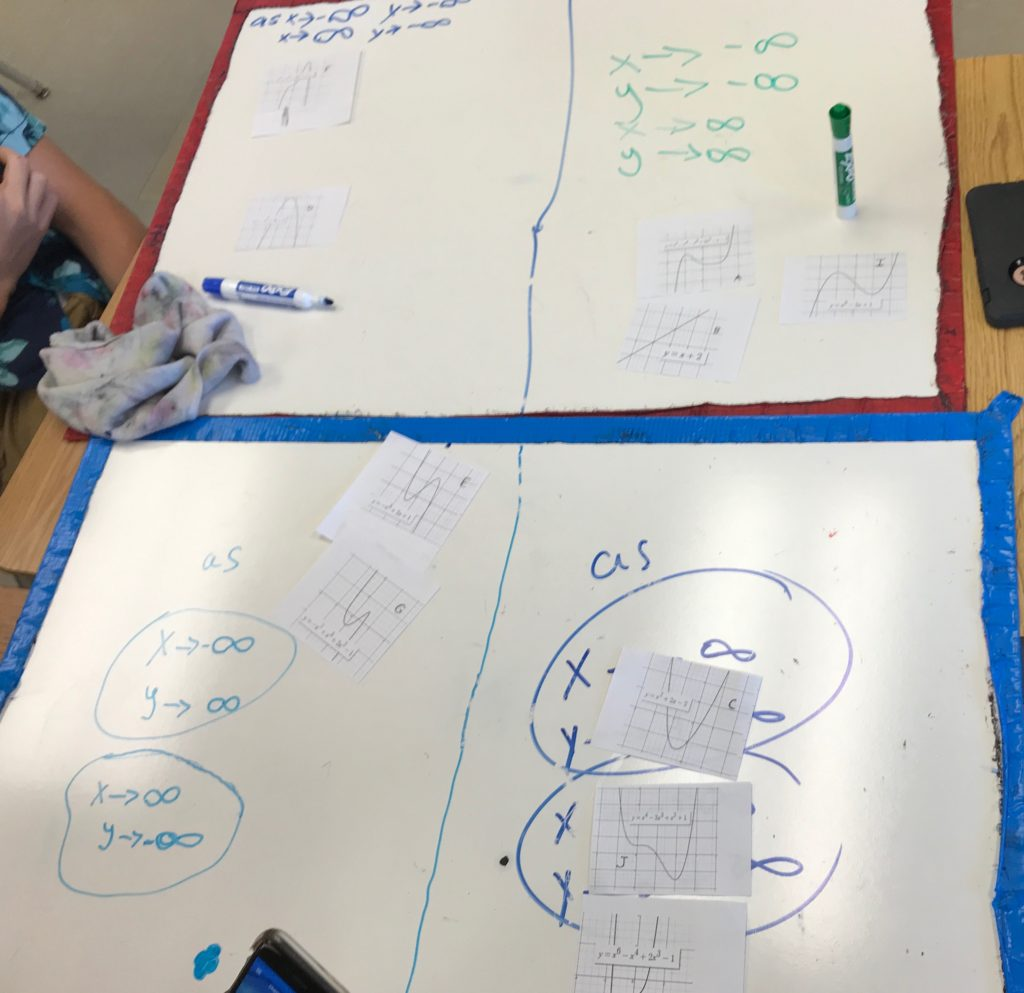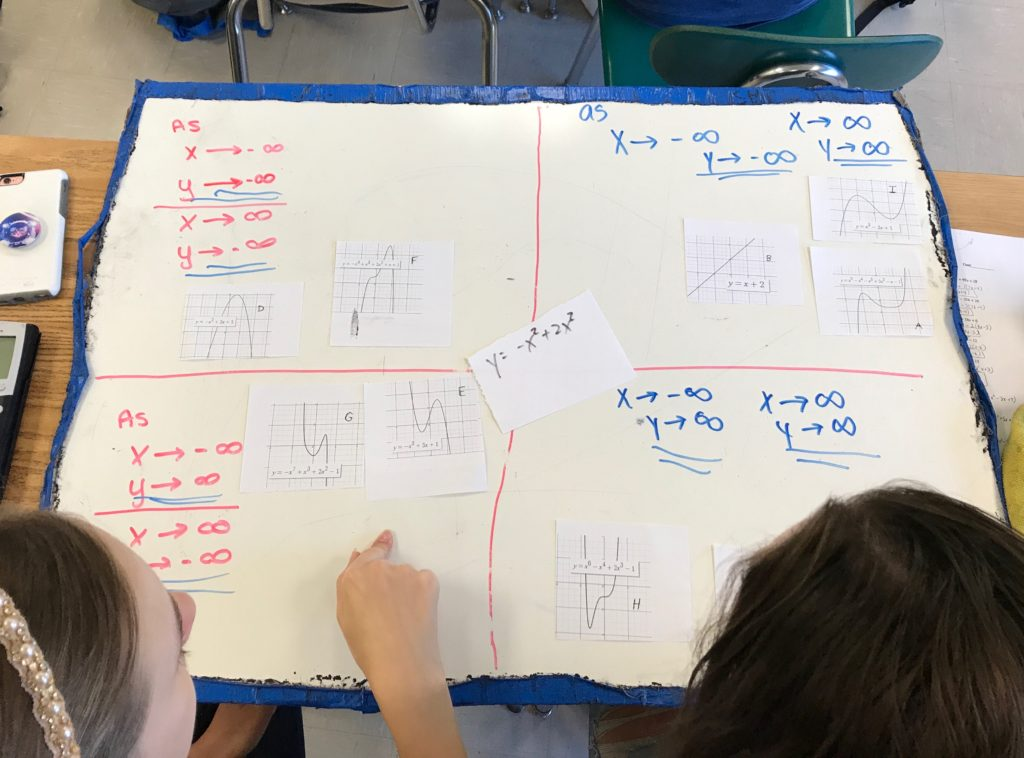As an introduction to end behavior of polynomials, I gave out the following pictures of graphs (with the equations pasted on top), and asked them to classify the graphs based on a set of conditions (for ex: as x -> -∞, y -> ∞ and as x -> ∞, y -> ∞).  After they had the graphs put into the categories (they were using the picture to classify), I asked them to find the similarities between the equations of each category. Every group recognized the negative or positive leading coefficient, but finding the second trait (the degree is even/odd) was difficult for them. As a test, I gave them the equation of one more graph and let them try to classify it by only the equation. This tweak (the first period didn’t have this addition) helped the groups figure out that the even/odd degree was a helpful piece of information.

I thought about doing this digitally, but I like what the student use of bits of paper added to the group discussion. I think I got more productive talk by using paper.